Cart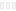Customer Support

#####0845 456 3089

7am - 8pm## How much do I need?!

Here at Dandy's we often get asked to help work out quantities needed for topsoil, bark, turf, gravel, slate and much more!

Here's some tips and advice to give you a helping hand, please note though these are just estimates and of course may not be exact - we advise you to under order so that you can always order more if you need to!

The basics...

Not everyone is a wizz at math but most of us will have a calculator on our mobile phones or tablets, doing the hard work for us!  Working out the area of a shape is the best start to working out how much product you need for your garden.

Rectangle or Square:

Width x Length = area

(We advise measuring in metres and cms but you can convert feet using our below equations).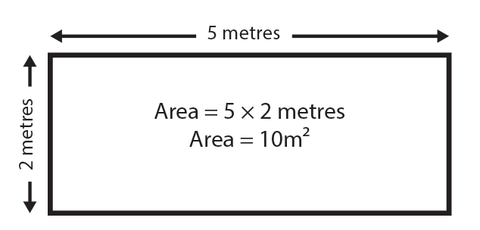Circle or Half Circle:

Circles are a little more tricky so follow these simple instructions and you should be fine!

We've all heard of (Pi r2) ... but if you're unfamiliar just bear with us!

The radius of a circle is the half way point so start off by measuring straight across at the largest point (the diameter).  Then divide this area by 2.  For example a diameter of 12m across gives a radius of 6m.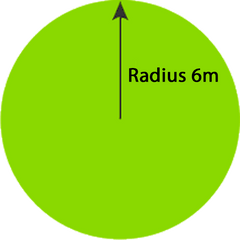So here's where is gets technical...

(Pi r2) is the equation.

We know that r = 6m by measuring the circle.  r2 = 6 x 6.  = 36m2

Pi = 3.14

3.14 x 36m = 113.04m2

Voila!

For half a circle just divide this area by 2.

Now you have the basics for a square of circle area you can start to figure out how much of each product you may need...

TIP:  If you've measured in feet simply work out the sq feet (using the same methods above) and then divide by 9 and then divide by 1.2  - This will give you the m2 (square metres).

Topsoil and Sand:

m2 (sq mtrs) x 1.2 x depth in inches, divide by 36 = TONNES you need.

1 x Jumbo Bag = 1 tonne

1 inch = 25mm or 2.5cmOrder Topsoil.

Order Sand.

Bark or Compost:

m2 x 0.05 = cubic metres you need per 2 inch depth (per 50mm/5cm)

1 x Jumbo Bag = 1 cubic metre

2 inch depth is the recommended minimum amountOrder Compost.

Order Bark.

Gravel and Slate:

m2 x 0.04 = TONNES you need per 1 inch depth

1 x Jumbo Bag = 1 tonne

2 inch depth is the recommended amount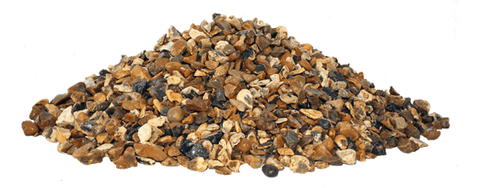Order Gravel and Slate.

Turf:

We sell turf by the m2 roll so simply work out how many sq mtrs you need and this is the amount of rolls.

25m2 = 25 x rolls

We advise taking extra for off-cuts, typically around 10%.

Order Turf.Paving:

We sell our Natural Stone Paving in packs of 18.9 m2.

If your area is less than this then unfortunately you'd need to just get the 1 pack.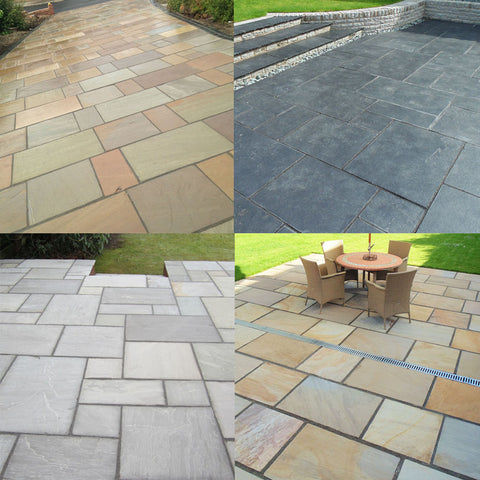Order Paving.

If you need any further help with working out how much you need please email us sales@dandystopsoil.co.uk or give us a quick call us on 01244 280008.